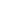MNIST的对抗实例：`import network.network as networkimport network.mnist_loader as mnist_loaderimport pickleimport matplotlib.pyplot as pltimport numpy as np`

`with open('trained_network.pkl', 'rb') as f:net = pickle.load(f)training_data, validation_data, test_data =mnist_loader.load_data_wrapper()`

`def predict(n):# Get the data from the test setx = test_data[n]# Get output of network and predictionactivations = net.feedforward(x)prediction = np.argmax(activations)# Print the prediction of the networkprint('Network output: ')print(activations)print('Network prediction: ')print(prediction)print('Actual image: ')# Draw the imageplt.imshow(x.reshape((28,28)), cmap='Greys')``def adversarial(net, n, steps, eta):"""net : network objectneural network instance to usen : integerour goal label (just an int, the function transforms it into a one-hot vector)steps : integernumber of steps for gradient descenteta : integerstep size for gradient descent"""# Set the goal outputgoal = np.zeros((10, 1))goal[n] = 1# Create a random image to initialize gradient descent withx = np.random.normal(.5, .3, (784, 1))# Gradient descent on the inputfor i in range(steps):# Calculate the derivatived = input_derivative(net,x,goal)# The GD update on xx -= eta * dreturn x``def sneaky_adversarial(net, n, x_target, steps, eta, lam=.05):"""net : network objectneural network instance to usen : integerour goal label (just an int, the function transforms it into a one-hot vector)x_target : numpy vectorour goal image for the adversarial examplesteps : integernumber of steps for gradient descenteta : integerstep size for gradient descentlam : floatlambda, our regularization parameter. Default is .05"""# Set the goal outputgoal = np.zeros((10, 1))goal[n] = 1# Create a random image to initialize gradient descent withx = np.random.normal(.5, .3, (784, 1))# Gradient descent on the inputfor i in range(steps):# Calculate the derivatived = input_derivative(net,x,goal)# The GD update on x, with an added penalty# to the cost function# ONLY CHANGE IS RIGHT HERE!!!x -= eta * (d + lam * (x - x_target))return x``def binary_thresholding(n, m):"""n: int 0-9, the target number to matchm: index of example image to use (from the test set)""" # Generate adversarial examplex = sneaky_generate(n, m)# Binarize imagex = (x > .5).astype(float) print("With binary thresholding: ") plt.imshow(x.reshape(28,28), cmap="Greys")plt.show()# Get binarized output and predictionbinary_activations = net.feedforward(x)binary_prediction = np.argmax(net.feedforward(x)) print("Prediction with binary thresholding: ")print(binary_prediction) print("Network output: ")print(binary_activations)`